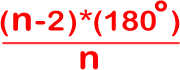METHOD 1:

Let's divide some regular polygons into triangles by connecting one vertex to all of the others...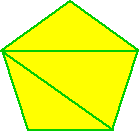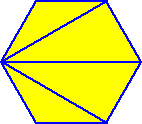A square has 4 sides and we made 2 triangles. A pentagon has 5 sides and we made 3 triangles. A hexagon has 6 sides and we made 4 triangles.

Do you see the pattern?

A heptagon has 7 sides... so we'd be able to make 5 triangles.

If we had polygon with n sides... we'd be able to make (n - 2) triangles.

Let's start with the square... We made 2 triangles. Notice that all of the interior angles of the 2 triangles make up the interior angles of the square.

The sum of the 2 triangle's angles is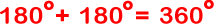There are 4 equal angles in a square,

sogives us that one angle of a square is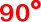!

Just what we expected.

Now for the pentagon.We made 3 triangles.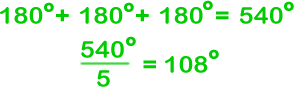So the interior angles of a regular pentagon are each 108 degrees.

Can you figure out the hexagon?How about a 100-gon? (That's a regular polygon with 100 sides.) There would be 98 triangles...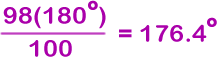So, in general, the measure of an interior angle of a regular n-gon is